#Function Repository Resource:

# FindGroupIsomorphism

Find up to a specified number of isomorphisms between two permutation groups

Contributed by: Daniel McDonald
 ResourceFunction["FindGroupIsomorphism"][g1,g2] finds an isomorphism between permutation groups g1 and g2. ResourceFunction["FindGroupIsomorphism"][g1,g2,n] finds up to n isomorphisms. ResourceFunction["FindGroupIsomorphism"][g1,g2,n,form] finds up to n isomorphisms, each having head form.

## Details and Options

The optional fourth argument form determines the format of each isomorphism returned.
The default value of form is List, which formats each isomorphism as a permutation list, where positive integer i being in position j indicates that the isomorphism sends the jth element of GroupElements[g1] to the ith element of GroupElements[g2].
If form has value Cycles, then each isomorphism is formatted as a disjoint cycle representation of its representative permutation list.
If form has value Association, then each isomorphism is formatted as an association whose key-value pairs are corresponding elements of g1 and g2 in the isomorphism.

## Examples

### Basic Examples

Find an isomorphism between two groups:

 In:=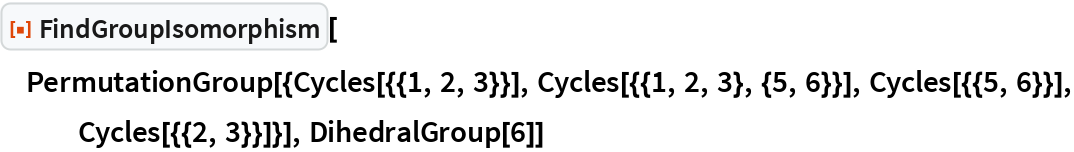Out=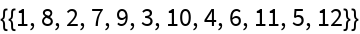Find three isomorphisms between two Abelian groups:

 In:=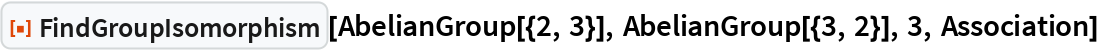Out=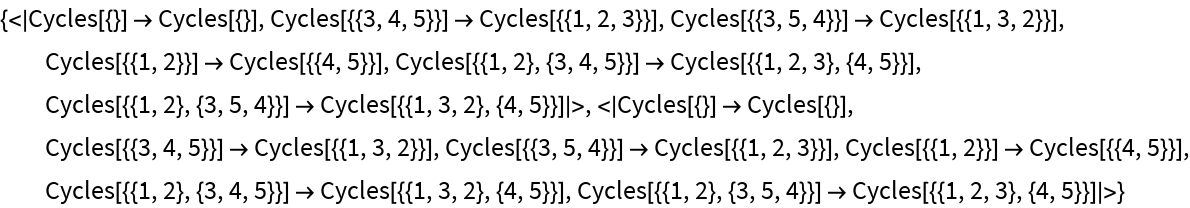Find all automorphisms of the symmetric group of degree four:

 In:=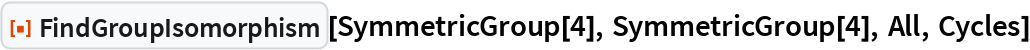Out=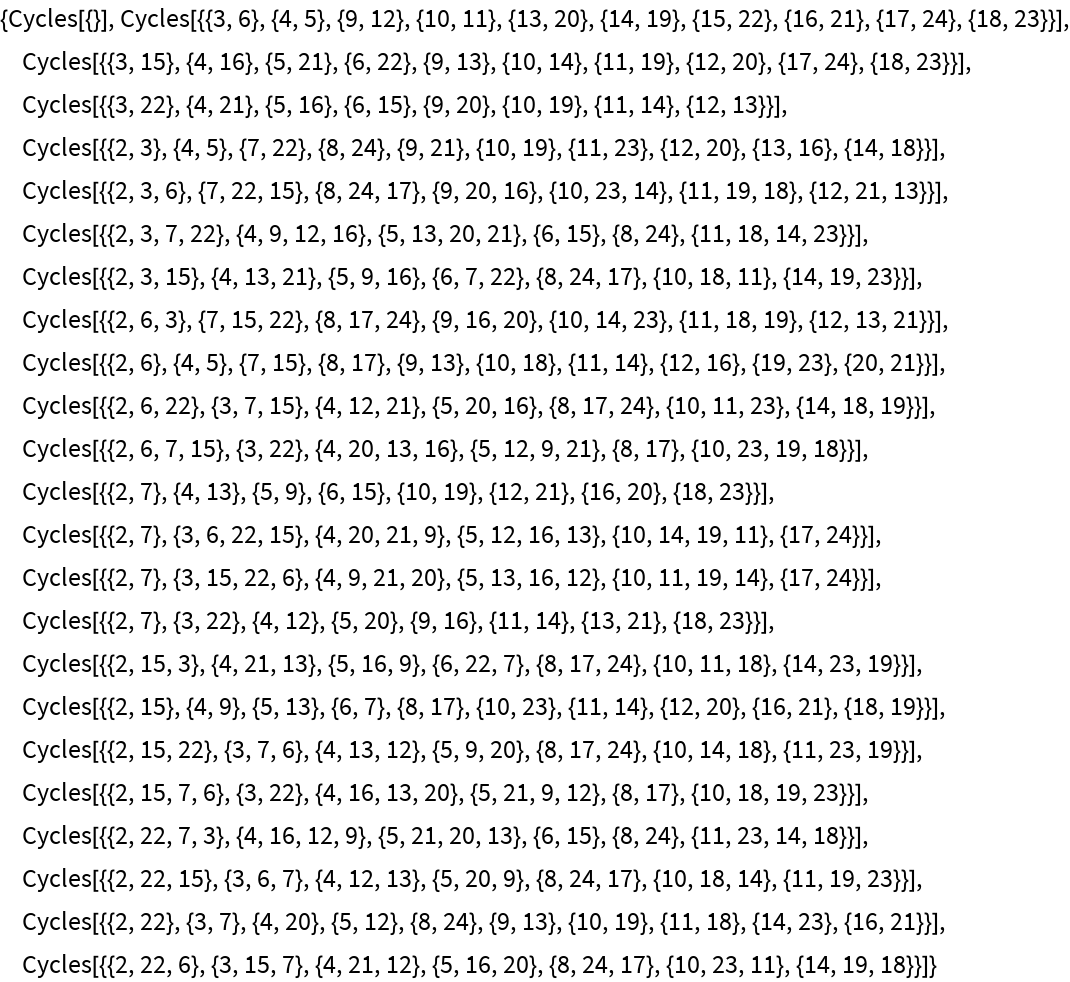### Scope

FindGroupIsomorphism works on groups with head PermutationGroup, SymmetricGroup, AlternatingGroup, CyclicGroup, DihedralGroup or AbelianGroup.

### Applications

Test if two groups are isomorphic by seeing if there is at least one isomorphism between them:

 In:=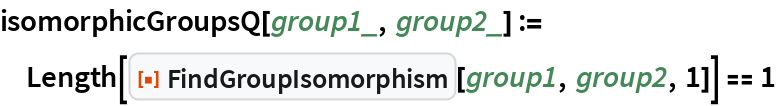See that the following two groups are isomorphic:

 In:=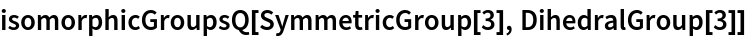Out=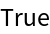See that the following two groups are not isomorphic:

 In:=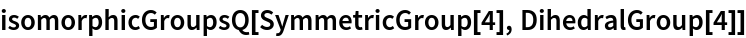Out=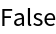Compute the automorphism group Aut(G) of a group G by finding all isomorphisms from that group to itself:

 In:=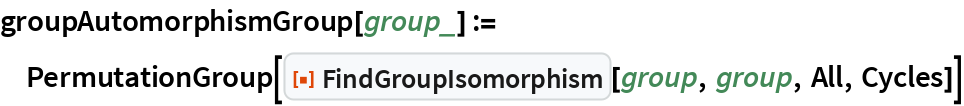Find the automorphism group of the following group:

 In:=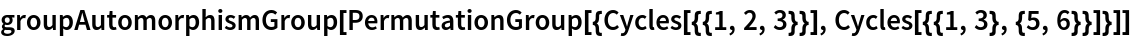Out=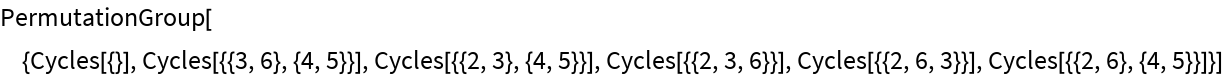### Properties and Relations

Format the isomorphism as a permutation list:

 In:=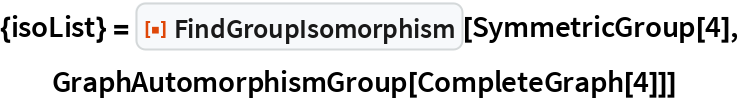Out=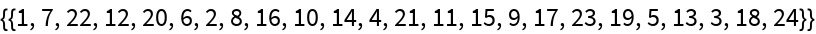Format the isomorphism as a disjoint cycle representation of its representative permutation list:

 In:=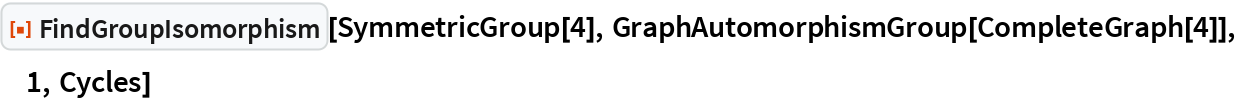Out=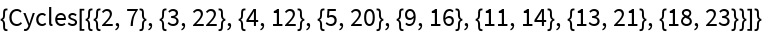This is equivalent to wrapping the permutation list representation of the isomorphism with PermutationCycles:

 In:=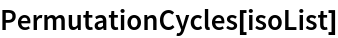Out=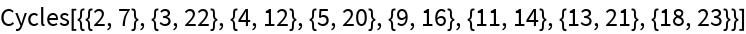Format the isomorphism as an association:

 In:=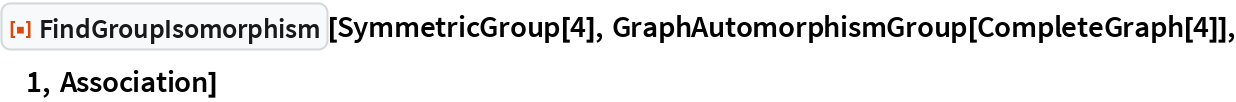Out=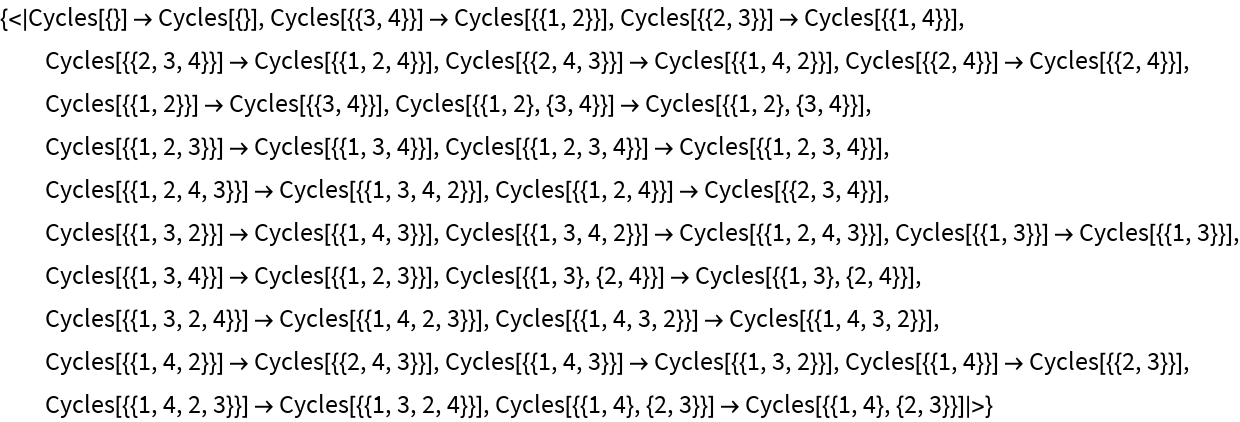This is equivalent to creating an association by threading the domain group elements to the permutation of the range group elements provided by the isomorphism:

 In:=Out=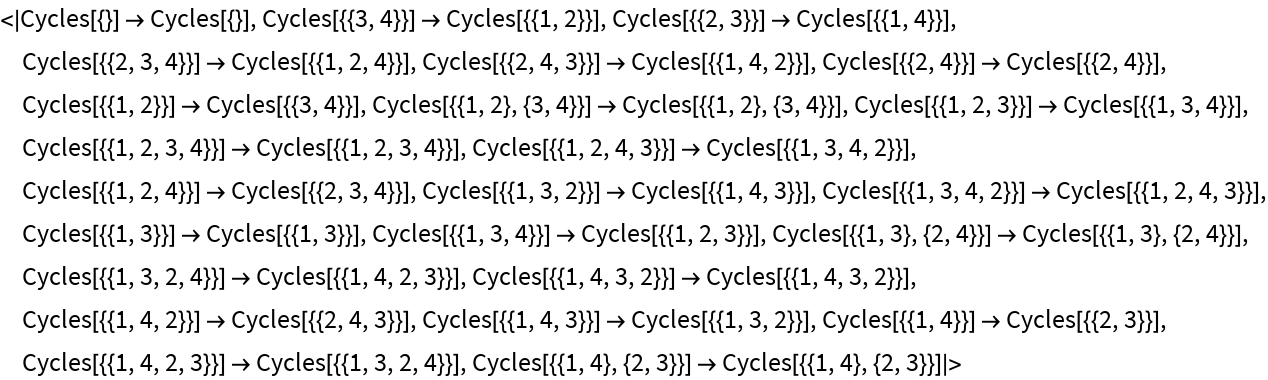## Requirements

Wolfram Language 11.3 (March 2018) or above